Hi you, and welcome the the third instalment in my blog series about my ongoing work! In the first post, I explained what time-stepping finite element analysis is and why it should be made faster in the first place. The second post basically discussed how the time-dependent FEM solution could be presented as a Fourier series – if only the coefficients could be calculated without having to generate a lot of samples in advance. So, enter the harmonic balance method.

The harmonic WHAT?

Sorry about the name. I didn’t come up with it.

Anyway, in the previous post I concluded that the time-dependent solution vector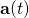to typical FEM problems could be written as a Fourier series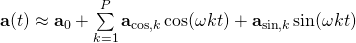.

By renaming the terms a bit, this can be more compactly written as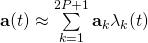,

where the time-function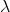is either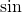or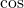, and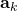are the corresponding Fourier coefficients.

No matter the notation, the number of terms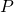could be quite reasonable, practically a small fraction of the number of samples produced by time-stepping analysis. Of course, the coefficientswould first have to be calculated.

And this is where the harmonic balance method steps in. Originally developed for analysing non-linear circuits, the method consists of writing the solution a Fourier series (non-surprisingly), and then solving the unknown coefficients. For this purpose, the power of the method of weighted residuals is harnessed.

### Galerkin’s method

…everybody calls it Galerkin’s method, though. This is technically correct, although sligthtly inaccurate, I guess.

Remember how the FEM problem was defined by a system of equations of form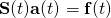?

The Galerkin’s method consists of first defining the residual – the difference between the two sides of the equation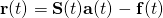.

The inner product of the residual and some suitable weight function is then set to zero.

Say what? More simply put, in this case, the residual is multiplied with a suitable function of time, e.g.. This yields us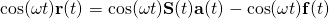.

Since this expression is still a function of time, the time-average is still calculated, and set to equal zero. In other words (or mathematical terms, to be exact), we get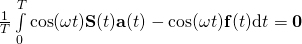.

For this, the more concise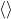is often adopted, so we can equivalently write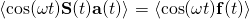.

This is often called the Galerkin’s orthogonality criterion.

### Matrix system

The last expression alone is of course meaningless, since we don’t know the solution. Thus, we finally substitute the Fourier series expression for, and so get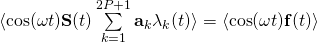Then, we take the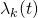functions of different frequencies that we have in the series, and in turn use each of them as a weight function in the orthogonality criterion. This, obviously, gives us as many equation-systems that we have unknown vectors. These can be expressed as a big block matrix system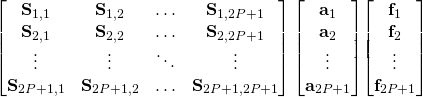,

with the blocks corresponding to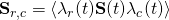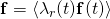.

By solving this system, we get the coefficients. And when we have the coefficients, we have an approximation for the time-dependent behaviouras.

### Results

And this finally brings us to the figure I have shown you two times already.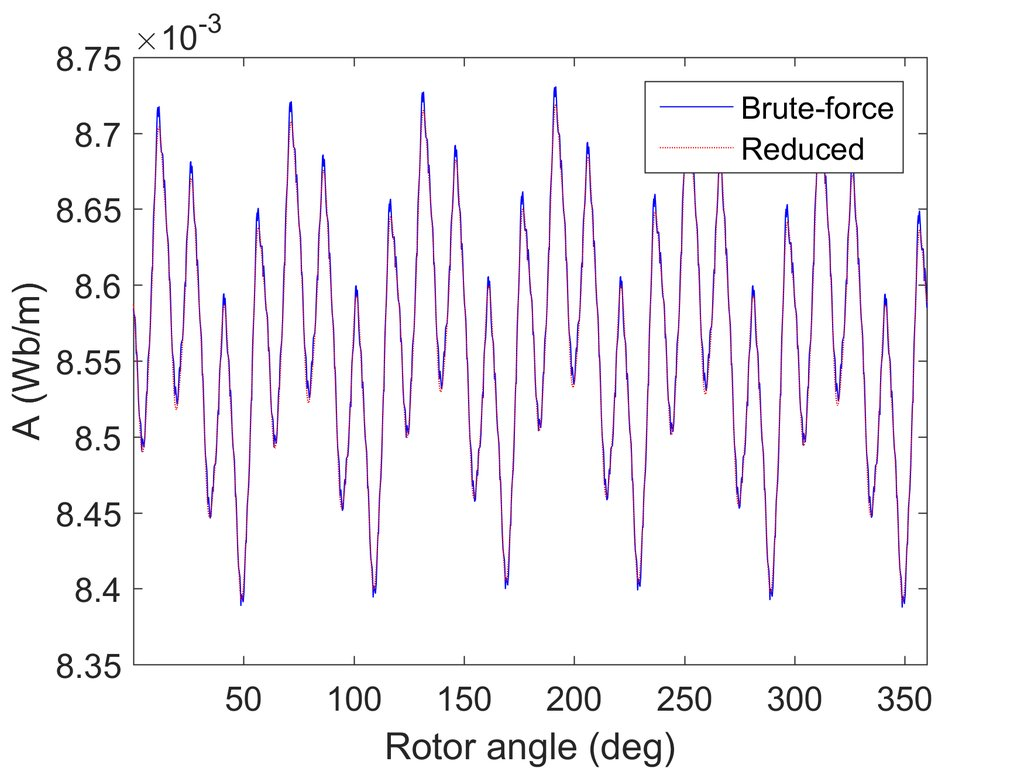Time-stepping results (brute-force) compared to the harmonic balance method (reduced).

The blue curve was obtained with nothing else than the harmonic balance method, with the number of terms equal to 15.

And as can be seen, the results are quite good indeed.

But, as often, they could still be improved. And I’m working on precisely that. But before that, let’s have one post about some practicalities!

Need help with electric motor design or design software? Let's get in touch - satisfaction guaranteed!Making time-stepping FEM faster, part 3